Skip to content

# Plotting Graphs

The easiest way to communicate information is through plotting a graph. Ggplot2, a library found in the tidyverse, contains tools that allow you to plot histograms, scatterplots, violin diagrams among other plots. You can gain an understanding of the trends and distributions of your data by utilizing this library.

## Example: Plotting and Configuring a Scatter Plot

In this example, we are going to be looking at the Cushings data from the MASS Package. Cushing’s syndrome is a disorder associated with the excessive secretion of cortisol from the adrenal gland. The data we are going to be looking at contains information about the excretory levels of two hormones Tetrahydrocortisone and Pregnanetriol in mg/24hr for 4 underlying syndromes of the disorder (adenoma, bilateral hyperplasia, carcinoma and unknown).

By analyzing the graphs, let’s try to understand the relationship between two hormones (do they increase proportionally to one another) and visualize the trends for each syndrome individually as well.

### The data frame

To access the data, you must load in the MASS library. As we will be creating some graphs, load in the ggplot2 library as well.

The first step it to take a look at the data frame itself.

```library(ggplot2)
library(MASS)
Cushings```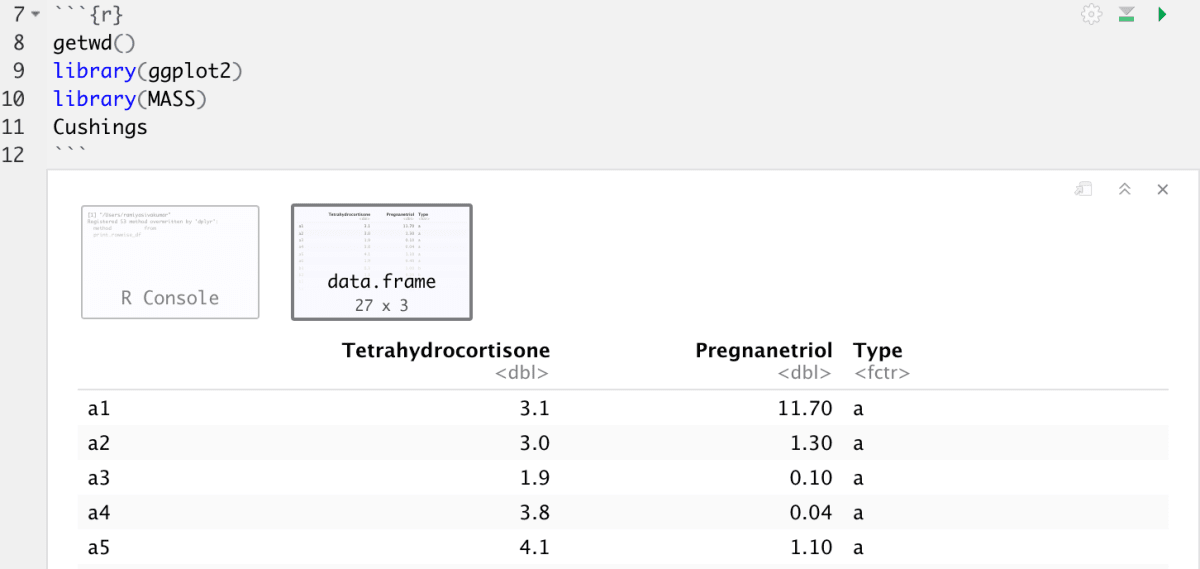The data contains 27 samples. There are 5-10 samples from each type of syndrome. For each sample, the excretory levels of Tetrahydrocortisone and Pregnanetriol are specified.

### ggplot

In this example, let’s plot the `Tetrahydrocortisone` values on the x-axis and the `Pregnanetriol` values on the y-axis.

The syntax looks like this:

` ggplot(data = Cushings) + geom_point(mapping = aes(x = Tetrahydrocortisone, y = Pregnanetriol))`

Let’s break this down:

• ggplot graphs begin with `ggplot(...`. This creates a coordinate system that you can add layers to.
• The first argument we provide to the function is the data: `ggplot(data=Cushings)`. If you were to plot this, you’d see a big gray box. This gray box is like a canvas. The data points are the paint.
• The function `geom_point()`adds a layer of points to your plot, which creates a scatterplot. ggplot2 comes with many geom functions that each add a different type of layer to a plot. Depending on which type of graph you are plotting, the input arguments’ syntax will vary.
• Each geom function in ggplot2 takes a `mapping` argument. This defines how variables in your dataset are mapped to visual properties. The `mapping` argument is always paired with `aes()`, and the `x` and `y` arguments of `aes()` specify which variables to map to the x and y axes. Ggplot2 looks for the mapped variable in the `data` argument, in this case, `Cushings`.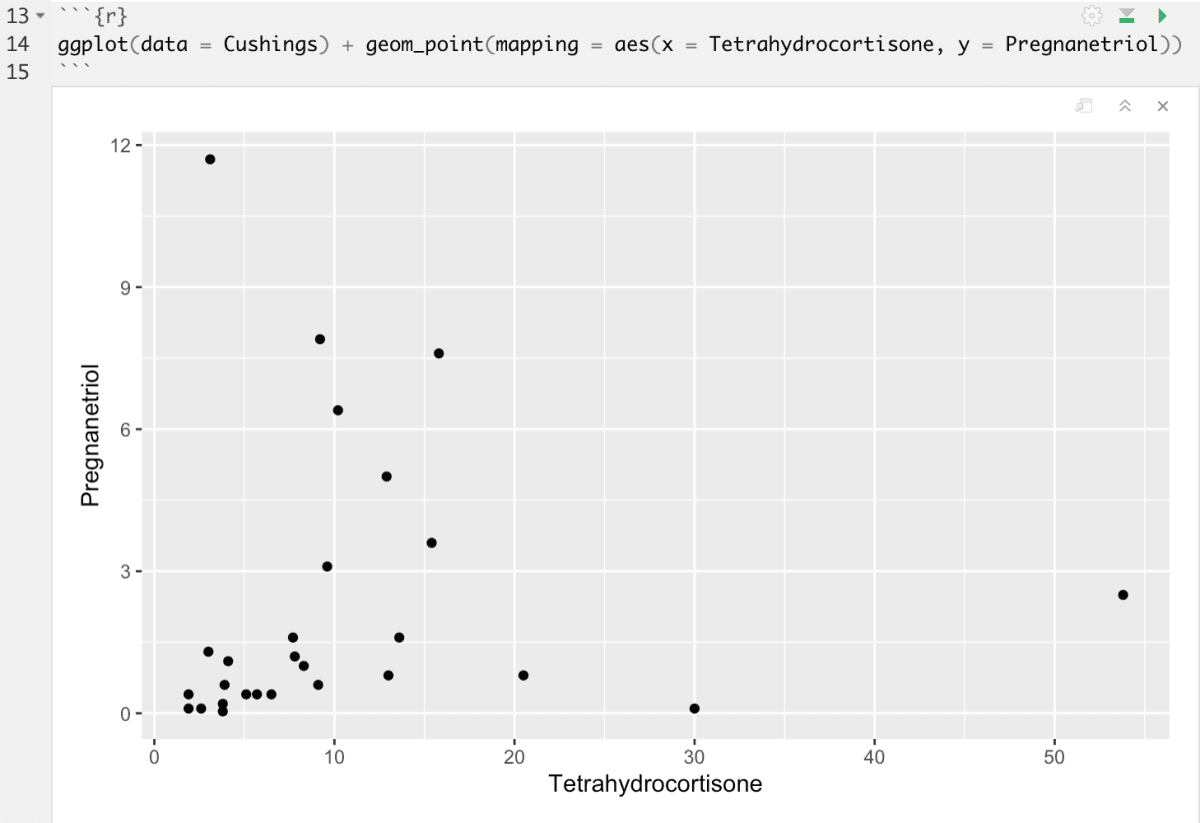The plot seems to show a positive correlation between the two parameters being tested. In other words, the secretion of the two hormones change proportionally to one another.

This graphing function is quite useful. Here is a general template for a mapping operation that you can modify according to the data you’d like to map.

`ggplot(data = <DATA> + <GEOM_FUNCTION>(mapping = aes(<MAPPINGS>))`

The rest of this chapter will show you how to complete and extend this template to make different types of graphs. We will begin with the `<MAPPINGS>` component.

My first thought is that I’d like some more information. How about we differentiate the data based on the type of underlying disorder.

You can add a third variable, like `type`, to a two dimensional scatterplot by mapping it to an aesthetic. An aesthetic is a visual property of the objects in your plot. Aesthetics include things like the size, the shape, or the color of your points. You can display a point (like the one below) in different ways by changing the values of its aesthetic properties. Essentially, we are categorically separating our data based on the type and visually displaying this by using the aesthetic property.

Since we already used the word “value” to describe data, let’s use the word “level” to describe aesthetic properties. We can visually represent our data levels as different kinds of points, colors, sizes, opacities and much more. You can convey information about your data by mapping the aesthetics in your plot to the variables in your dataset.

Looking back at our dataset, I can see that the type parameter can take on 4 levels. differentiating the type based on color might be a good way to show differentiation.

`ggplot(data = Cushings) + geom_point(mapping = aes(x = Tetrahydrocortisone, y = Pregnanetriol, color = class))`

within the `aes()` , I add `color = Type` to tell the script to make each type a different color.ggplot2 will automatically assign a unique level of the aesthetic (here a unique color) to each unique value of the variable, a process known as scaling. Ggplot2 will also add a legend that explains which levels correspond to which values.

In the above example, we mapped `Type` to the color aesthetic, but we could have mapped `Type` to the size aesthetic in the same way. In this case, the exact size of each point would reveal its class affiliation.

`ggplot(data = Cushings) + geom_point(mapping = aes(x = , y = , size = Type))`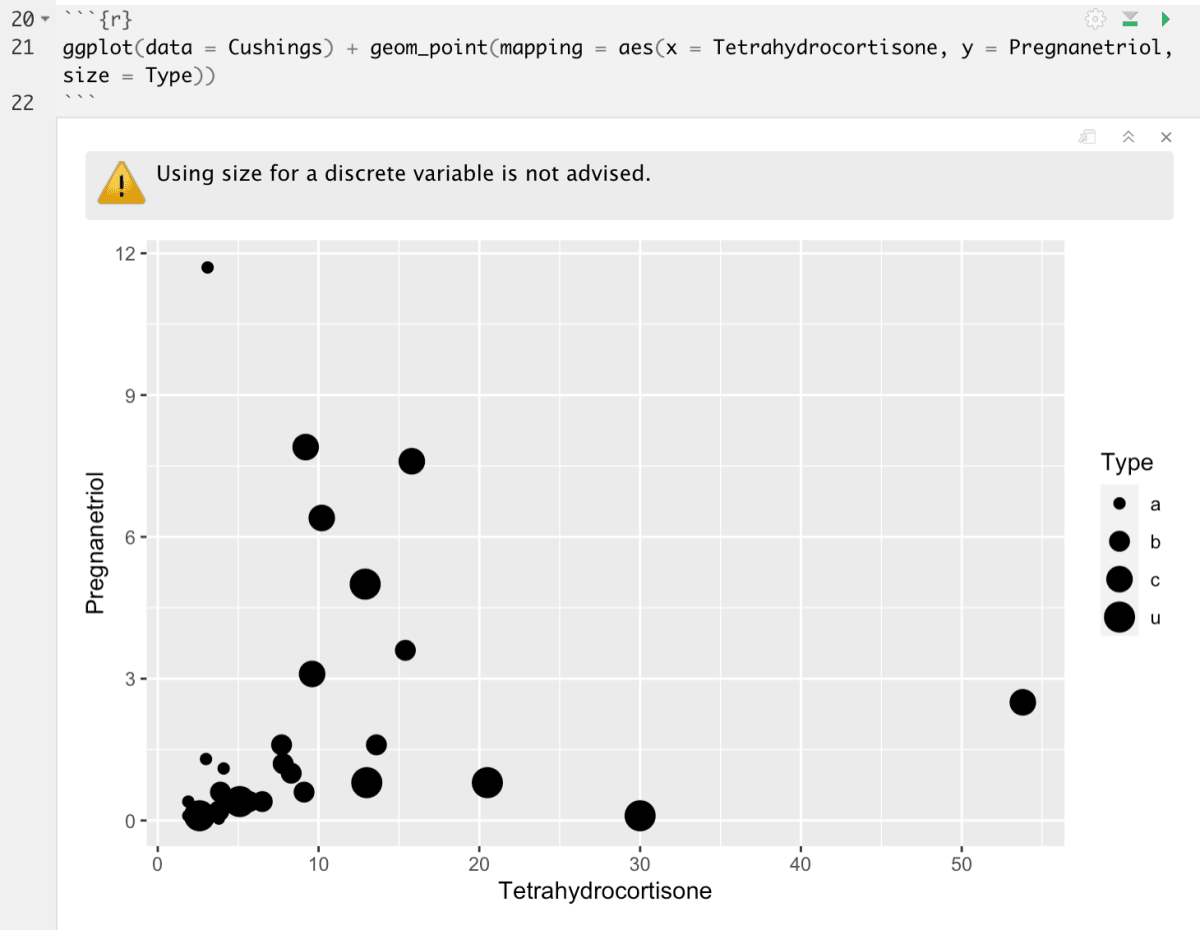We get a warning here, because mapping an unordered variable (`Type`) to an ordered aesthetic (`size`) is not a good idea.

Mapping `Type` to the alpha aesthetic, controls the transparency of the points.

`ggplot(data = Cushings) + geom_point(mapping = aes(x = , y = , alpha = Type))`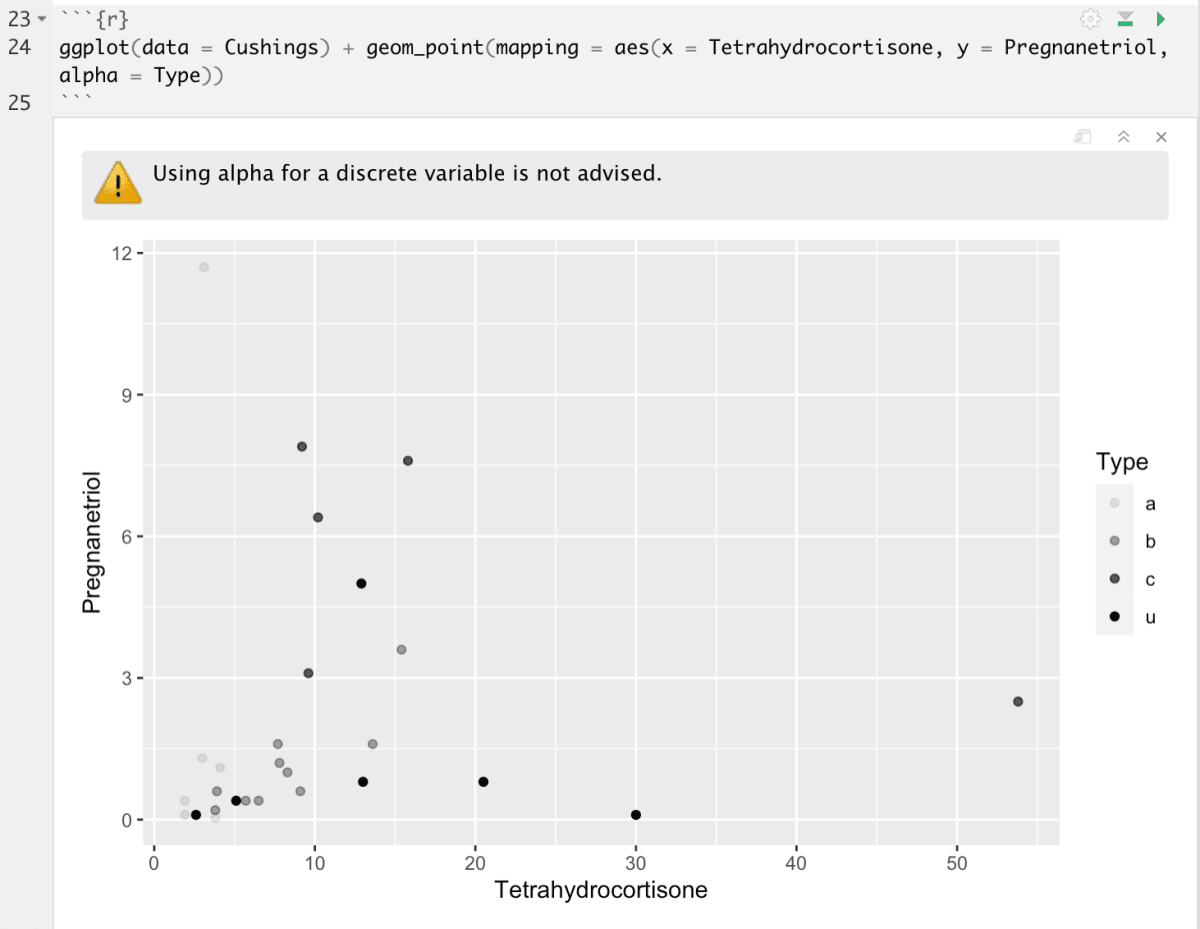Again, we get the same warning because alpha is also an non-discrete method of plotting the points. This is just something to keep in mind when choosing your method of differentiating classes of a variable.

The shape aesthetic changes the shape of each point.

`ggplot(data = Cushings) + geom_point(mapping = aes(x = , y =, shape = Type))`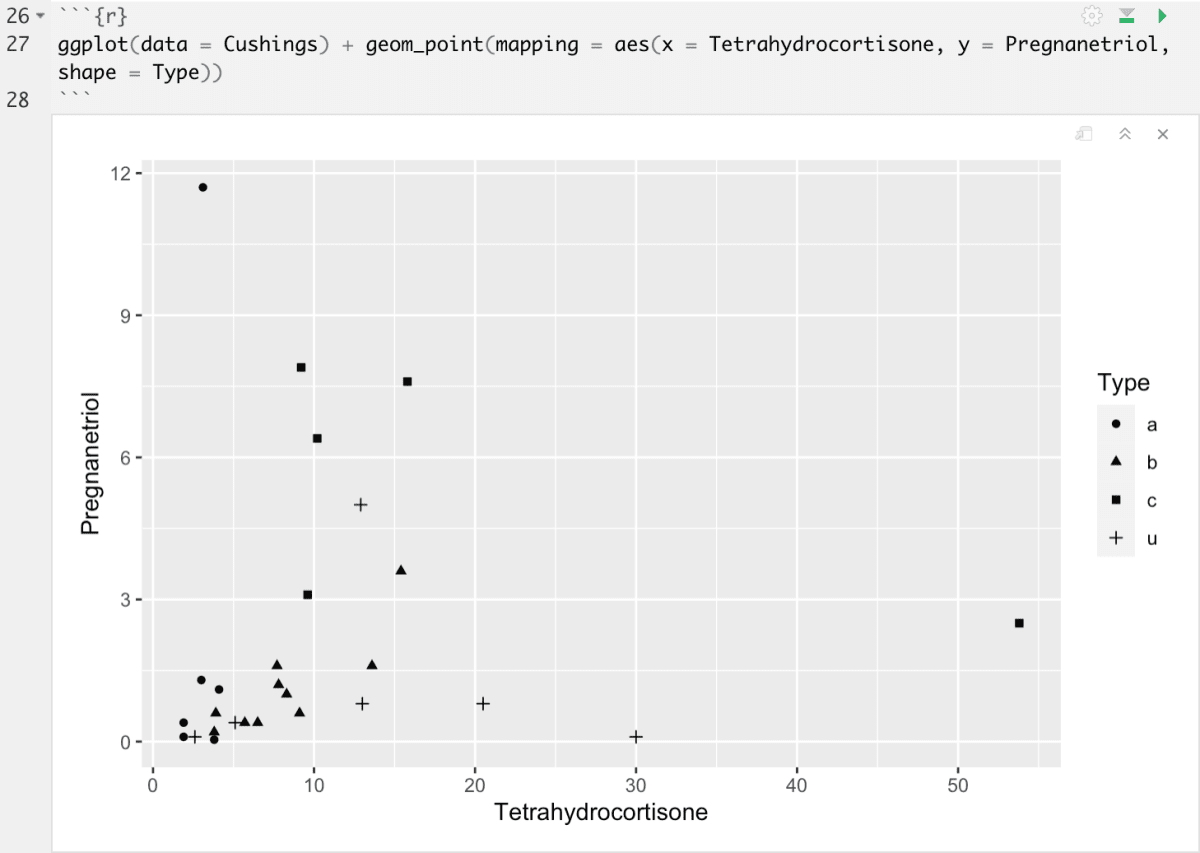Note: when using the shape property, you can only include a variable that has 6 or less levels. The aesthetic will omit other levels.

 Aesthetic Function: Note color levels are differentiated by color size levels are differentiated by sizing unordered, not advised for unordered levels alpha levels are differentiated by opacity shape levels are differentiated by various shapes maximum 6

The `aes()` function gathers together each of the aesthetic mappings used by a layer and passes them to the layer’s mapping argument. The syntax highlights a useful insight about `x` and `y`: the x and y locations of a point are themselves aesthetics, visual properties that you can map to variables to display information about the data.

Once you map an aesthetic, ggplot2 takes care of the rest. It selects a reasonable scale to use with the aesthetic, and it constructs a legend that explains the mapping between levels and values. For x and y aesthetics, ggplot2 does not create a legend, but it creates an axis line with tick marks and a label. The axis line acts as a legend; it explains the mapping between locations and values.

### Modifying the Visuals of the Whole Graph:

Above, we used the `aes()` property to change the property based on a level for each point. Now, let’s look at some way to modify the overall graph to better suit our vision.

`geom_point` takes `color` and `size` as arguments that modify ALL the points in the plot in the same way. Here is an example of the usage.

`ggplot(data = Cushings) + geom_point(mapping = aes(x = Tetrahydrocortisone, y = Pregnanetriol), color = "cadet blue", size=4)`

Notice that the `color = "cadet blue"` argument is not within the `aes()` function, but it is still within `geom_point`.To add labels to the plot (in this case I want to add the units), we can use the `labs` function (this is being added succeeding the existing lines of script):

`ggplot(data = Cushings) + geom_point(mapping = aes(x = Tetrahydrocortisone, y = Pregnanetriol, color = Type)) + labs(x="Tetrahydrocortisone(mg/24hr)", y="Pregnanetriol(mg/24hr)")`To change the theme of the plot, you can add the `theme_`function. The prompt should suggest some options. I’ve opted for an option that draws defined lines on the borders of my plot.

`ggplot(data = Cushings) + geom_point(mapping = aes(x = Tetrahydrocortisone, y = Pregnanetriol, color = Type))+ labs(x="Tetrahydrocortisone(mg/24hr)", y="Pregnanetriol(mg/24hr)")+ theme_linedraw()`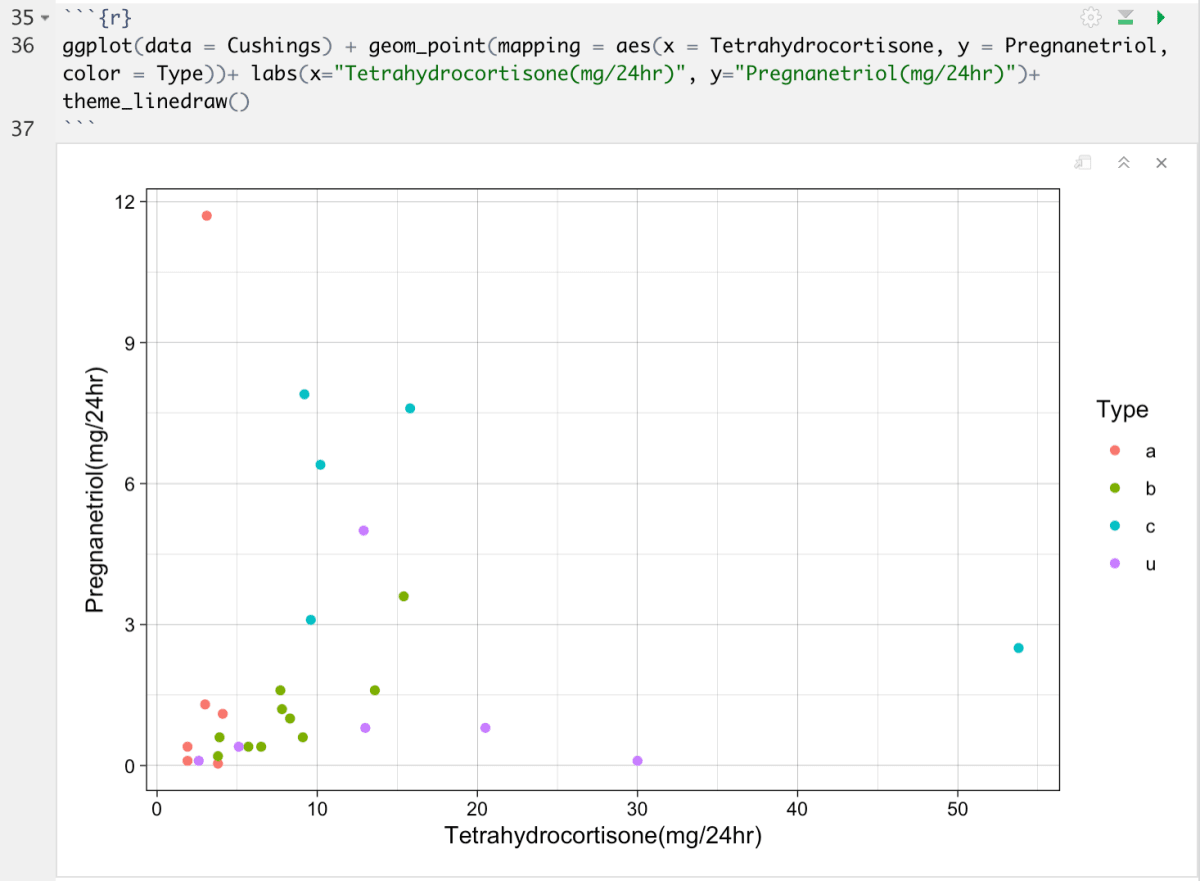Summary Table of Visual Modifications:

 Argument Function Syntax Example Color of Points Changes the color of points `color = "cadet blue"` Size of Points modifies size of points `size =4` Title Add a table to the top of the graph `labs(Title="Example")` Axis Labels Modifies the default axis title `labs(x="Tetrahydrocortisone (24 mg/hr)")` Theme Changes the theme of the plot `theme_dark()`

### Other type of graphs:

You can plot many types of graphs using the ggplot library. Similar to geom_point which plots a scatter plot, functions such as geom_bar and geom_violin can be used to plot a bar graph and violin plot respectively.